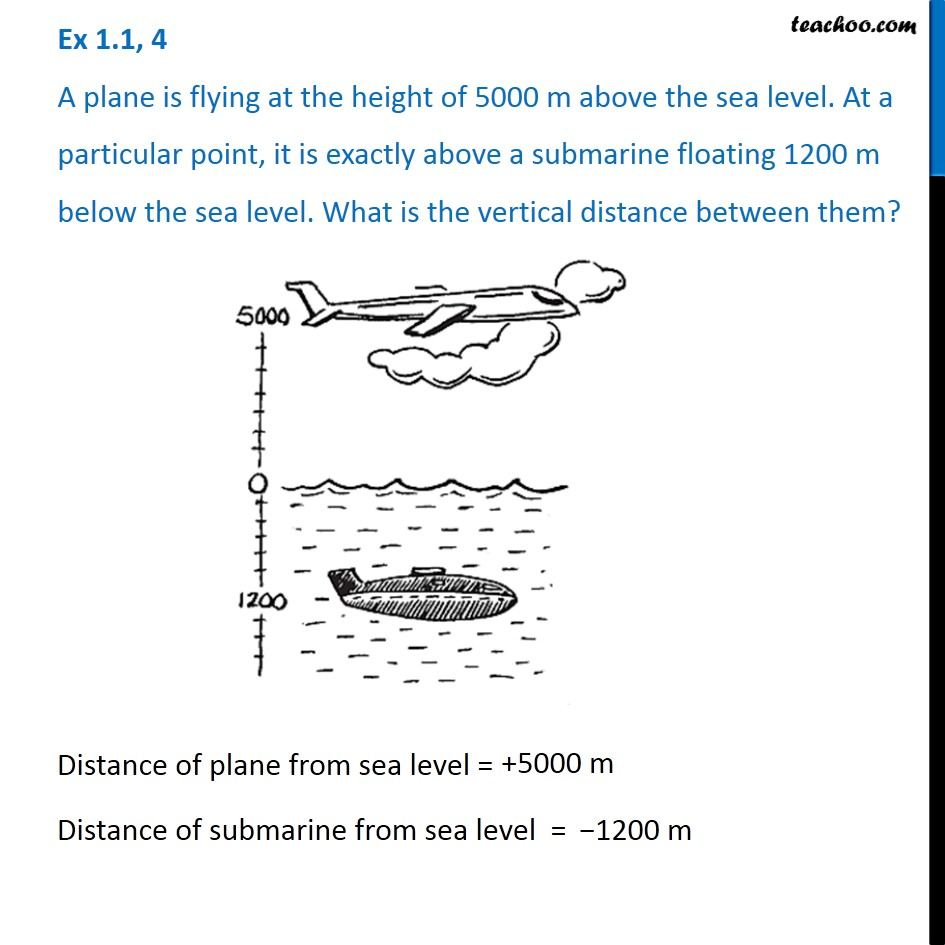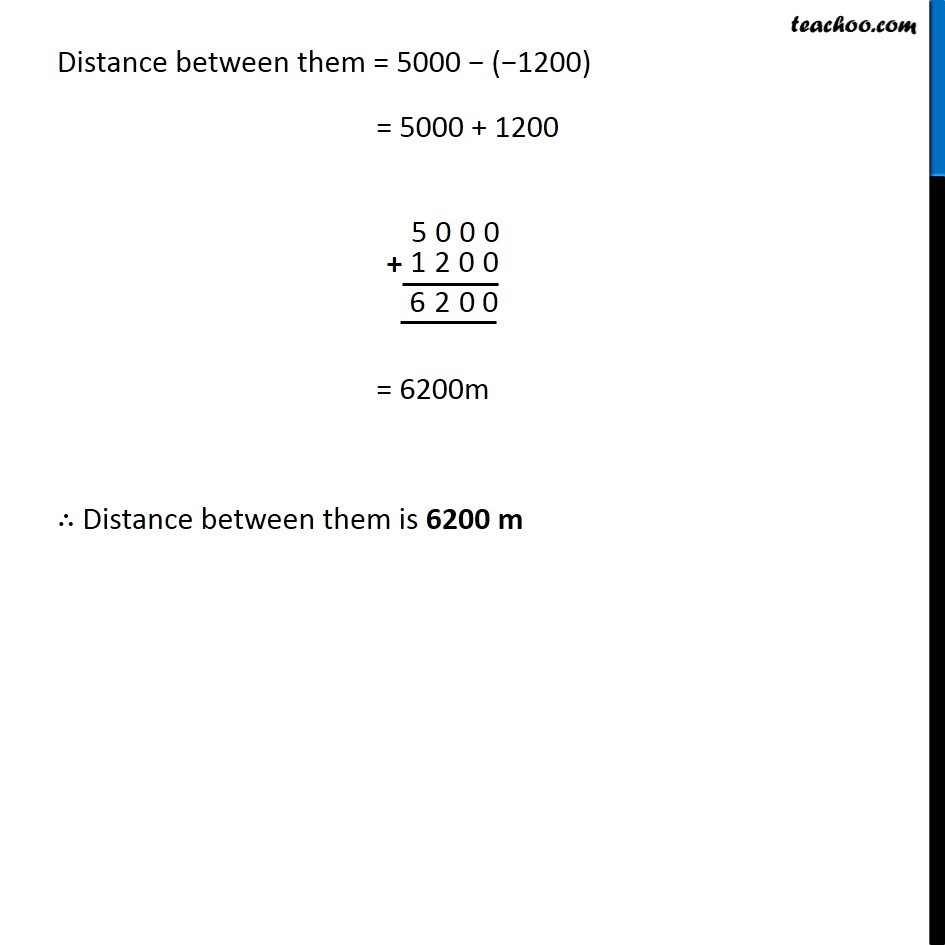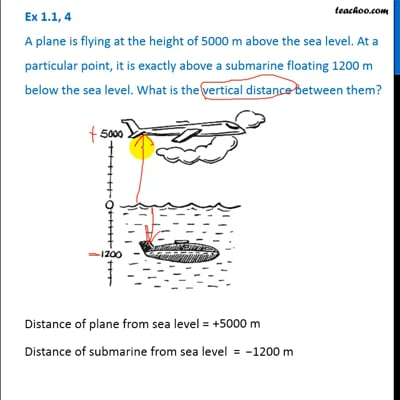Ex 1.1

Chapter 1 Class 7 Integers
Serial order wiseThis video is only available for Teachoo black users

Introducing your new favourite teacher - Teachoo Black, at only ₹83 per month

### Transcript

Ex 1.1, 4 A plane is flying at the height of 5000 m above the sea level. At a particular point, it is exactly above a submarine floating 1200 m below the sea level. What is the vertical distance between them? Distance of plane from sea level = +5000 m Distance of submarine from sea level = −1200 m Distance between them = 5000 − (−1200) = 5000 + 1200 = 6200m ∴ Distance between them is 6200 m